Courses

# Worksheet 2 - Measurement Notes | EduRev

## Class 1 : Worksheet 2 - Measurement Notes | EduRev

The document Worksheet 2 - Measurement Notes | EduRev is a part of the Class 1 Course Mathematics for Class 1: NCERT.
All you need of Class 1 at this link: Class 1

Q.1. Circle the object that weights more.
A.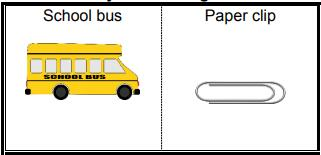B.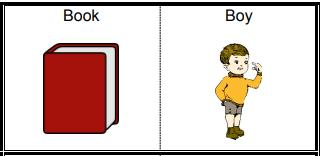C.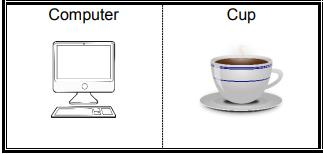D.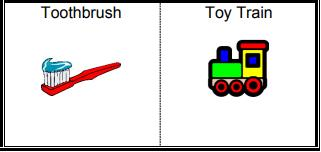Ans.
A.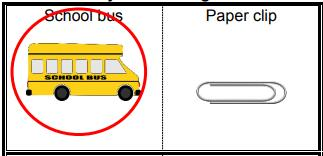B.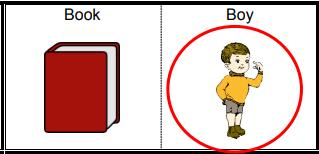C.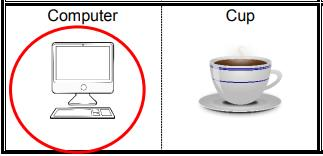D.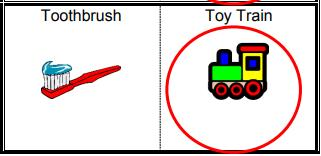Q.2. Circle the object that weights less.
A.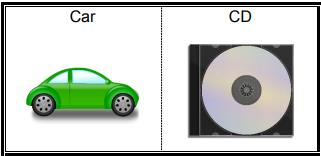B.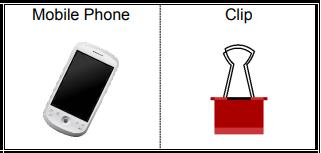C.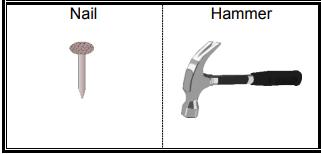D.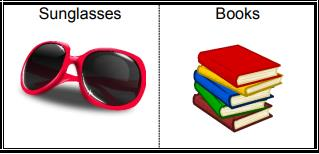Ans.
A.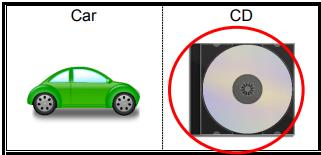B.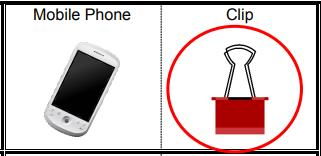C.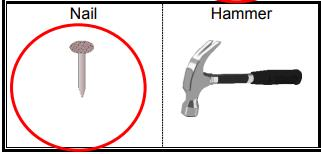D.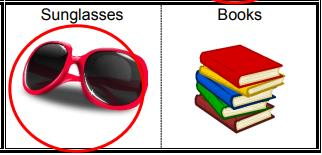Q.3. Circle the objects that weights more.
A.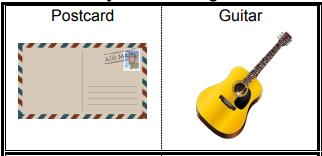B.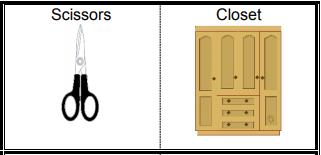C.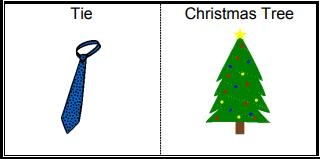D.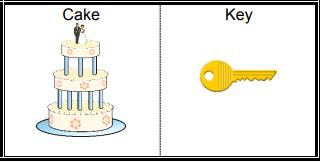Ans.
A.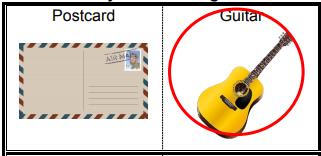B.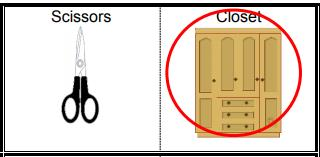C.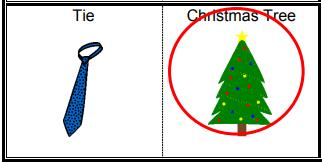D.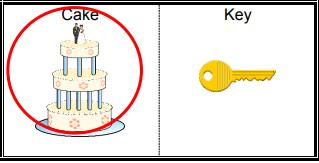Q.4. Circle the objects that weights less.
A.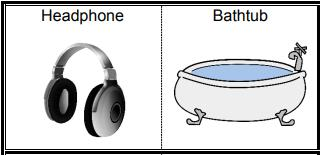B.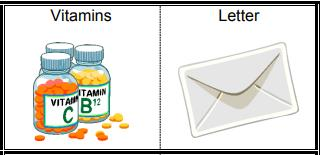C.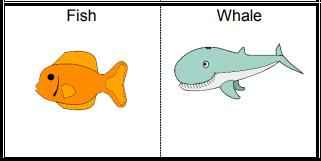D.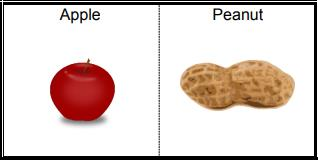Ans.
A.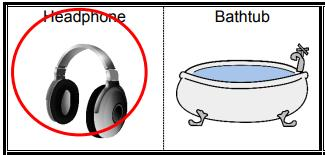B.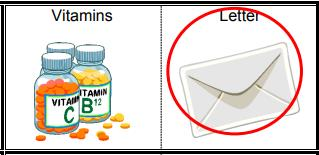C.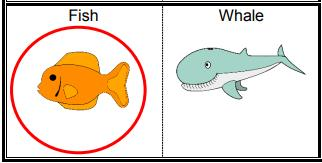D.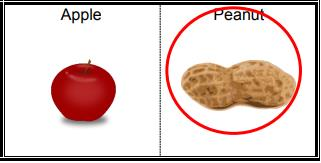Q.5. Circle the objects that weights more.
A.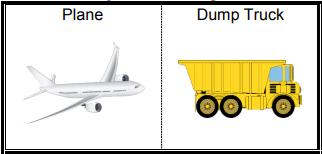B.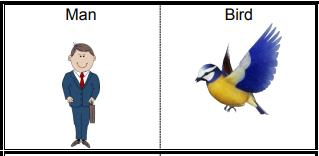C.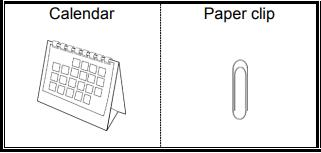D.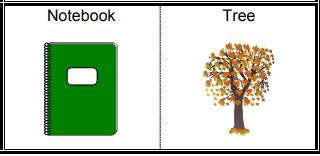Ans.
A.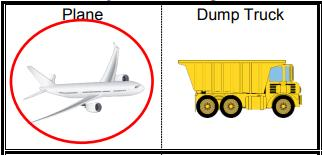B.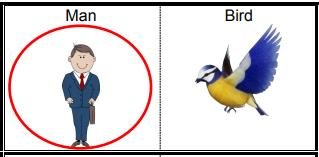C.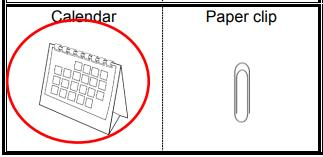D.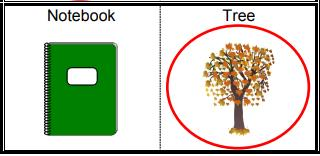Q.6. Circle the objects that weights less.
A.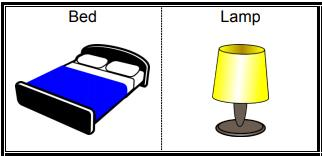B.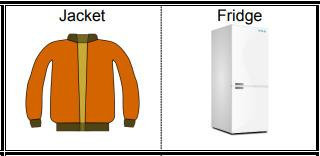C.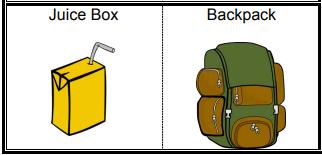D.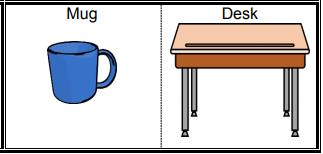Ans.
A.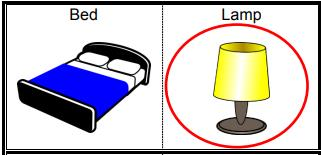B.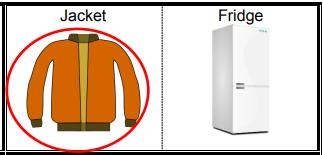C.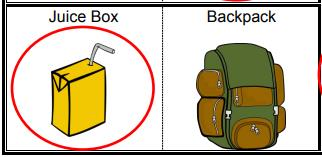D.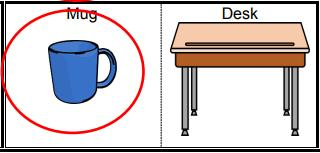Q.7. Circle the objects that can hold more.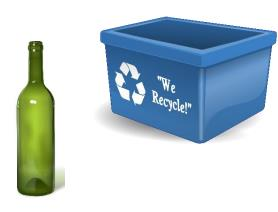Ans.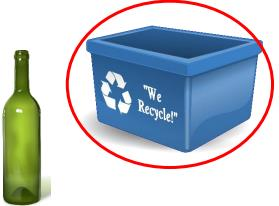Q.8. Circle the objects that can hold more.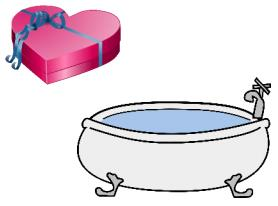Ans.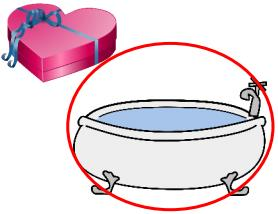Q.9. Circle the objects that can hold more.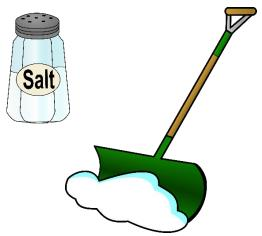Ans.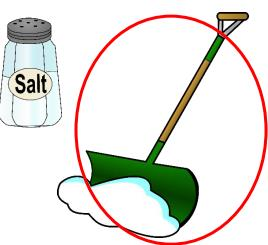Q.10. Circle the objects that can hold more.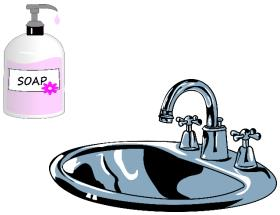Ans.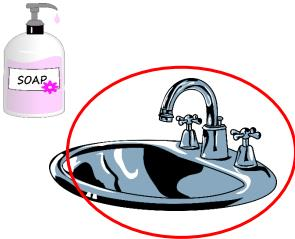Q.11. Circle the objects that can hold more.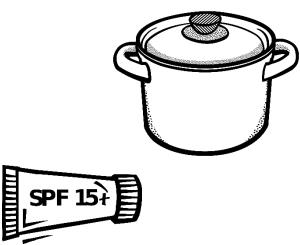Ans.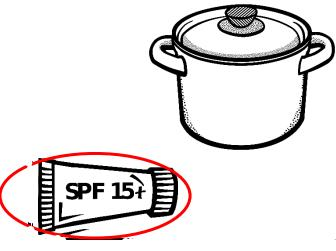Q.12. Circle the objects that can hold less.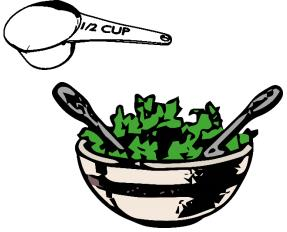Ans.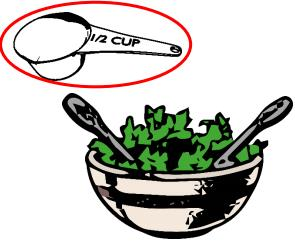It’s arts and craft time for Jacob and his little brother Sean.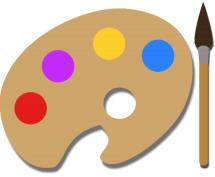Q.13. Jacob’s scissors are 7 inches long. Sean’s scissors are 2 inches shorter than Jacob’s. How long are Sean’s scissors?
Ans. 7 - 2 = 5
Sean’s scissors are 5 inches long.

Q.14. Their mother gives the boys two pieces of strings to use. Sean’s string is 8 inches long and Jacob’s is 5 inches long. What is the total length of their strings?
Ans. 8 + 5 = 13
The total length is 13 inches.

Ashley is helping her sister, Emily, to do her hair. Ashley has three pieces of ribbon: a 15-inch long red ribbon, an 8-inch long yellow ribbon and a 12-inch long purple ribbon.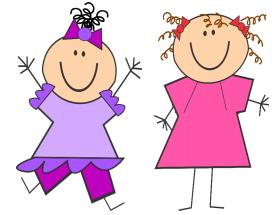Q.15. Which piece of ribbon is the shortest?
Ans. The yellow ribbon is the shortest.

Q.16. Compared to the red one, how much shorter is the yellow one?
Ans. 15 – 8 = 7
The yellow one is 7 inches shorter than the red one.

William is picking a bookshelf for his room. There is a brown shelf that is 15 inches tall, a white shelf that is 12 inches tall and a black shelf that is 20 inches tall.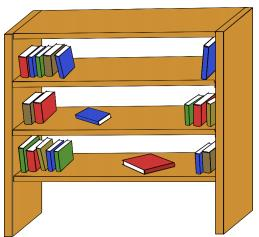Q.17. Which shelf is the tallest?
Ans. The black shelf is the tallest.

Q.18. If he stacks the brown shelf on top of the white shelf, how tall will the shelves be?
Ans. 15 + 12 = 27
The shelves will be 27 tall.

Emma has three rulers: a white one that is 15 cm long, a yellow one that is 25 cm long and a brown one that is 10 cm long.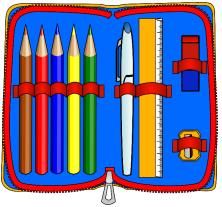Q.19. Which ruler is the shortest?
Ans. Brown ruler

Q.20. Compared to the shortest ruler, how much longer is the longest ruler?
Ans. 25 – 10 = 15
The longest ruler (yellow) is 15 cm shorter than the shortest ruler (brown).

William has three stacks of cards. The stack of white cards is 20 cm thick. The stack of black cardstocks is 24 cm thick. The stack of brown cardstocks is 12 cm thick.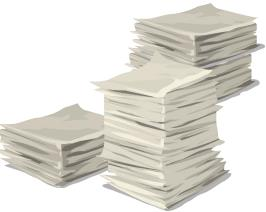Q.21. Which stack of cards is the thinnest?
Ans. Brown cards

Q.22. Compared to the white cards, how much thicker is the stack of black cards?
Ans. 24 – 20 = 4
The stack of black cards is 4 cm thicker than the stack of white cardstocks.

Sean has a box of building blocks. The blue blocks are 3 cm tall. The red blocks are 6 cm tall and the yellow blocks are 2 cm tall.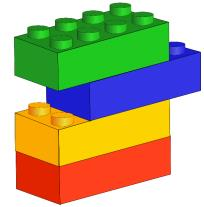Q.23. Which kinds of blocks are the tallest?
Ans. Red blocks

Q.24. Compared to a yellow block, how much taller is a red block?
Ans. 6 – 2 = 4
A red block is 4 cm taller than a yellow block.

Q.25. If a blue block is stacked on top of a red block, how tall are the two blocks?
Ans. 3 + 6 = 9
The two blocks are 9 cm tall.

Offer running on EduRev: Apply code STAYHOME200 to get INR 200 off on our premium plan EduRev Infinity!

## Mathematics for Class 1: NCERT

52 videos|43 docs|25 tests

,

,

,

,

,

,

,

,

,

,

,

,

,

,

,

,

,

,

,

,

,

;